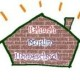# Year 2 Maths Curriculum | Spring Term

Below are the Year 2 Maths lesson plan ideas for Spring term 2019.1. Time
– Tell and write the time to 5 minutes including quarter past/to the hour, half past and o’clock.
– Know the number of minutes in an hour and hours in a day.2. Fractions:
– Recognise, find and name a half (1/2), quarter (1/4) or third (1/3) of an object/shape or set of objects.
– Understand the non unit fractions 2/4 and 3/4.3. Addition and subtraction:
– Add/subtract numbers using concrete objects, pictures and mentally; including a two-digit number and ones (TU+U), a two-digit number and tens (TU+T), two two-digit numbers (TU+TU), T: tens U: units/ones.

4. Money:
– Recognise and use symbols for pounds (£) and pence (p).
– Combines coins to make a particular value.
– Find different different combinations of coins that equal the same amounts of money.
– Calculate change to be given.

5. Faces, shapes and patterns:
– Identify number of sides and lines of symmetry in 2D shapes.
– Identify and describe the properties of 3D shapes including number of edges, vertices and faces.
– Sequence of shapes.
– Describe position, direction and movement including left turn, right turn, clockwise and anticlockwise.

For non-commercial, education use only.